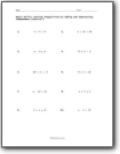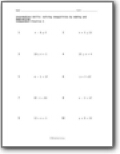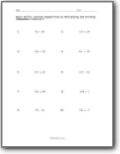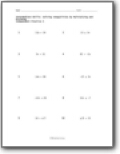# Solving Inequalities Worksheets

#### What are Inequalities?

There are three types of mathematical functions that students have to deal with. There are expressions, which combine constants, variables, and arithmetic operations. Then some equations combine all three elements with an equals-to sign. Then there are inequalities, and these combine constants, variables, and arithmetic operations along with an inequality sign. Inequality signs include greater-than, less-than, greater-than-or-equal-to, and less-than-or-equal-to. They are constantly used to describe and bring order to a mathematical relationship. They are posed like questions or math statements. They can often be solved much like equations.###### Solving Inequalities by Adding and Subtracting

If you treat the comparison symbol much like a equal symbol in an equation, they are easy to solve.###### Variable Inequalities

These all involve a single move on your part.###### Solving Inequalities by Multiplying and Dividing

Treat them as if they were equations.###### Products and Quotients When Solving Inequalities

You will work with negative values, at times.